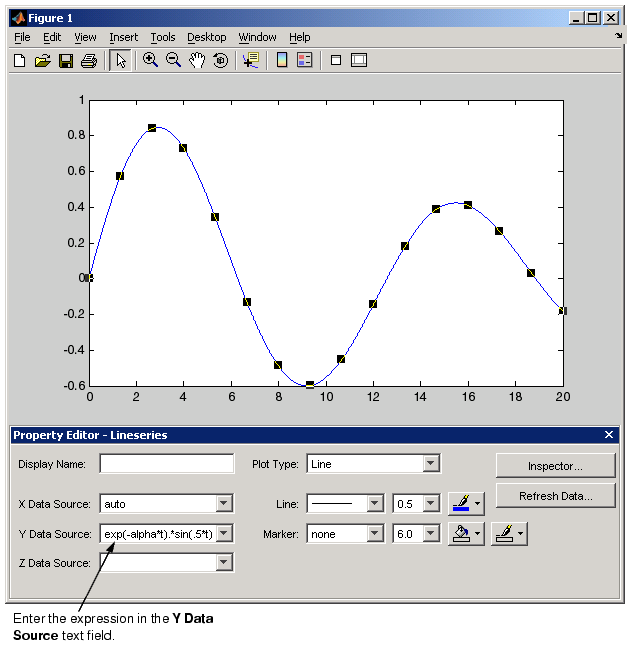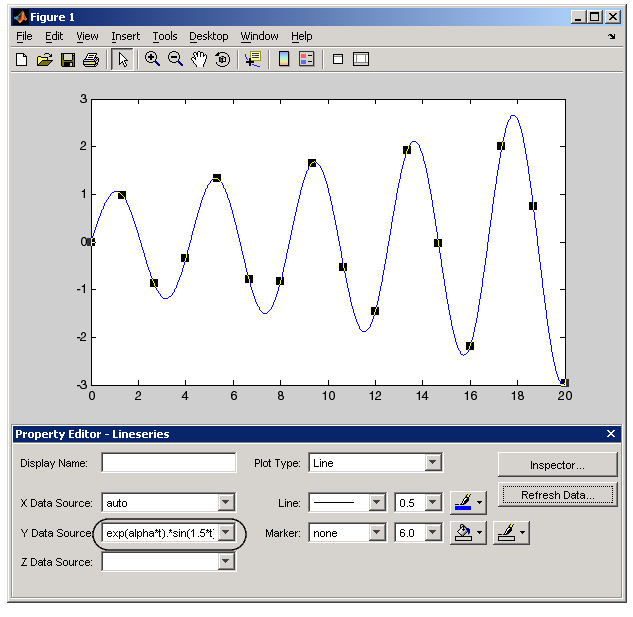GraphicsExample -- Specifying a Data Source

Plot objects have properties that enable you to specify the source of the data that defines the object. For example, you can specify a workspace variable name or a MATLAB expression as the value of the `XDataSource`, `YDataSource`, or `ZDataSource` property for a line in a plot (i.e., a lineseries object). You can then use the Property Editor to change the variable name or alter the expression, and the plot is updated to reflect the change.

Creating the Graph

First define two variables by issuing these statements in the command window.

• ```t = 0:.01:20;
alpha =.055;
```

Next plot `t` vs. the expression `exp(-alpha*t).*sin(.5*t)` using the `plot` function or the plot tools.

• ```plot(t,exp(-alpha*t).*sin(.5*t))
```

Varying the Data Source

After creating the graph, you can use the Property Editor to couple the plotted line to the MATLAB expression.

1. Double-click on the plotted line to display its property panel.
2. Enter the MATLAB expression in the Y Data Source text field.You can now modify the expression in the Y Data Source text field and observe how the graph changes. After changing the text, click the Refresh Data button to update the data.

In the following picture, `alpha` is no longer negated, so the function grows instead of decays. Also the period has been shortened by changing `sin(.5*t)` to `sin(1.5*t)`.Data Sources for Multiobject Graphs

Suppose you create a line graph from matrix data. For example,

• ```z = peaks;
h = plot(z,'YDataSource','z');
```

Because MATLAB creates one lineseries object for each column of `z`, the following is true.

The data source for `h(1)` is `z(:,1)`.

The data source for `h(2)` is `z(:,2)`.

• ``````...
``````

The data source for `h(n)` is `z(:,n)`.Example -- Plotting Workspace Variables Example -- Generating M-Code to Reproduce a Graph© 1994-2005 The MathWorks, Inc.